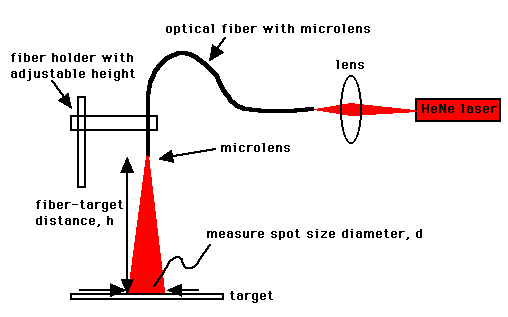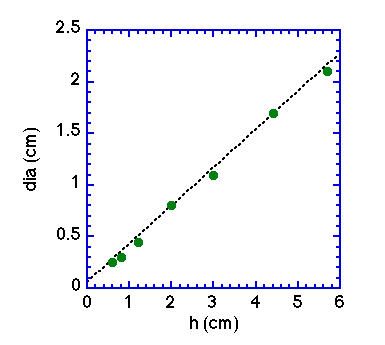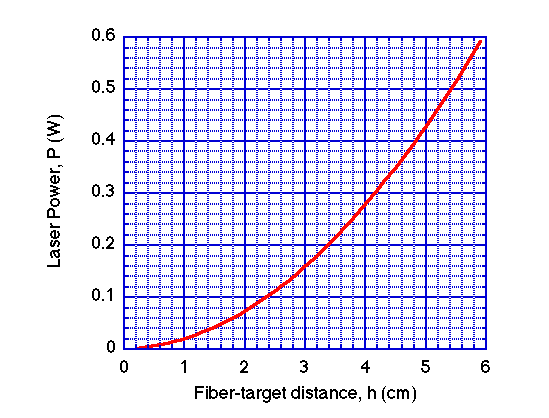# Calibration of microlens fiber.

The spot size of laser light on the target tissue depends on the fiber-target distance. So in the clinic, the doctor must adjust the laser power for a particular fiber-target distance to yield the desired irradiance of 0.150 W/cm2. For use in the dosimetry protocol of this article, we needed to determine how the spot size of the microlens fiber depended on the fiber-target distance, and use that information to set the laser power. Here is how we determined the calibration graph used in our dosimetry protocol.

A microlens fiber was purchased from Rare Earth Medical Inc.. In the lab prior to the clinical treatment, a HeNe laser (633 nm) was launched into the fiber. The fiber microlens terminus was positioned above a flat white paper at variable heights h (cm) and the diameter d (cm) of the spot size was determined. Hence, the function h(d) was specified, as shown below.Set up for measuring spot size d versus fiber-target distance h.Plot of spot size diameter d versus fiber-target distance h.

For a given fiber-target distance h, the graph (above right) specifies a spot diameter d on the target tissue. The irradiance E is specified by the laser power P (Watts) divided by the area of the laser spot (cm2):

E = Power/Area = P/(pi (d/2)2)

Rearranging, the appropriate power setting is:

P = E((pi (d/2)2)

The desired E is 0.15 W/cm2. The above expression was used to generate a curve relating the required laser power setting P (W) to the fiber-target distance h, as shown below:

## CALIBRATION GRAPHThe required laser power setting P as a function of the fiber-target distance h in order to achieve the desired irradiance E = 0.15 W/cm2. (This calibration is for a particular microlens fiber.)

Back to article | August NewsEtc | NewsEtc Contents page